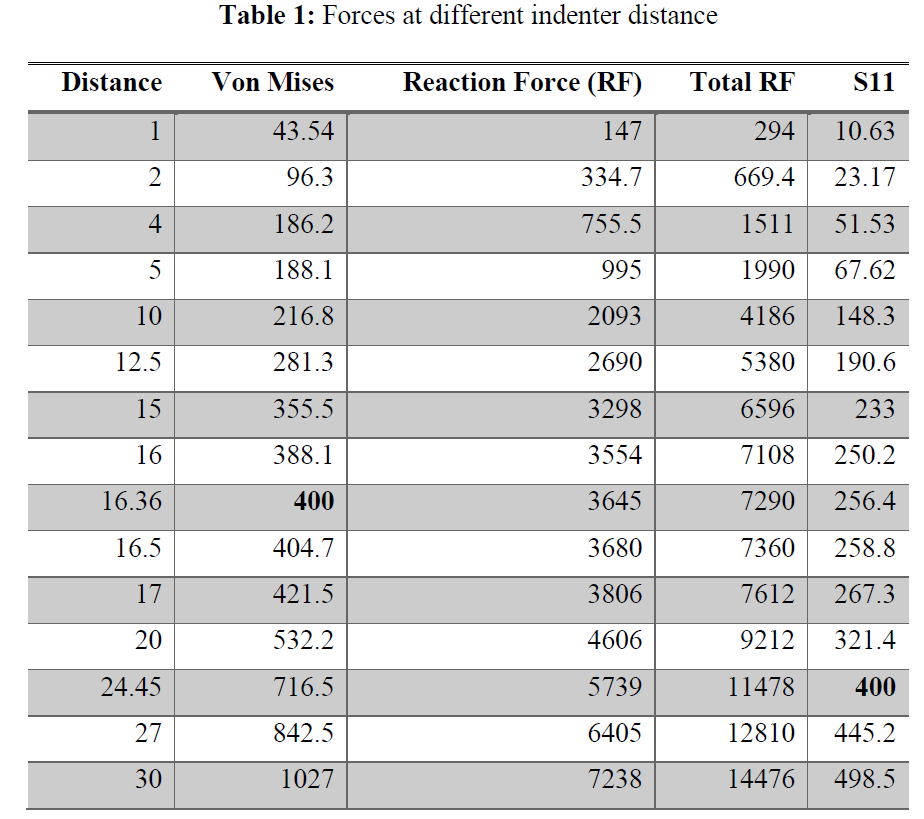## Welcome to the World of Modelling and Simulation

### What is Modelling?

This blog is all about system dynamics modelling and simulation applied in the engineering field, especially mechanical, electrical, and ...### Dynamics of Contact Simulation in ABAQUS

This problem describes a configuration, where a hollow cylinder is squeezed between a fixed flat indenter and a cylindrical indenter where force will be applied to see the fracture in the hollow cylinder. The inside and outside radii of the hollow cylinder are 30 mm and 100 mm respectively. The material of the hollow cylinder has a Young’s modulus of 2000 Mpa, and a Poisson’s ratio of 0. This problem is solved by Abaqus using the following steps.

Fig. 1: Showing a hollow cylinder rests on a flat indenter where load is applied on it by a cylindrical indenter on top.

## Creating the Geometry:

Fig. 1 shows the hollow cylinder where it sits on a fixed rigid indenter, and a for is applied by the cylindrical indenter on top. There parts are required to be created. At first, in Abaqus CAE, the geometry of the hollow cylinder consisting two circles is created. The half of it is then trimmed since we will be implementing symmetry boundaries on its geometry. Then a cylindrical indenter is drawn by a half circle where the property is specified as an analytically rigid wire. The flat indenter is created similarly. Two reference points (RPs) are specified on the cylindrical and flat indenter accordingly, which are seen in Fig. 2. In this problem, part-1 is the cylindrical indenter, part-2 is the hollow cylinder, and part-3 is the flat indenter.

Fig. 2: Showing the overall geometry as described in Fig. 1.

## Selecting Material Property:

The material property is specified for the hollow cylinder according to the problem.Fig.3: Assigning the material properties to the hollow cylinder.

## Meshing the Part:

The hollow cylinder is meshed using approximate global size 10 and seeding the edges. Plain strain is used, and reduced integration is applied for the 4 node linear meshing elements. Also, a partition is created on the surface of the cylindrical indenter to apply loads on the hollow cylinder.

Fig. 4: Meshing the hollow cylinder.

## Applying Boundary Conditions:

After meshing is done, next we move forward with the boundary conditions. Two steps are created. In the first step, five boundaries (BCs) for the geometries are created. BC-1 and BC-2 are the symmetry boundary conditions for the hollow cylinder. BC-3 is the fixed symmetry boundary for the flat indenter, which is applied at the reference point. BC-4 is applied to the RP of the cylindrical indenter that is a displacement boundary of 50 mm in the downward direction to help facilitate the loading. BC-5 is another displacement boundary, which is applied to the other end of the hollow cylinder to restrict its horizontal movement.
Interactions are created to represent the contact between the hollow cylinder and indenter. The surface-to-surface contact mode is applied, where the tangential and normal mechanical behaviors are chosen. The friction coefficient is set to 0.2, and the penalty method is selected for the constraint.

Fig. 5: Applying boundary conditions to the geometries.## Simulation Results:

The simulation is performed with the application of different indenter displacements. After applying considerable amount of forces, we see that the stress concentration occurs at the edges inside of the hollow cylinder. Fig. 6 depicts the location of the failure.

Fig. 6: Results showing the failure location.

We see that the distance at which the failure will occur is at 16.36 mm (When 400 Mpa Von Mises), and the load is 7.29 KN (Twice the reaction force).

The following graph shows the relation between the force and displacement.

The following figure shows the distance at which the Von Mises stress will reach 400 Mpa.

#Blog #Blogger #FiniteElementMethod #Abaqus #ContactDynamics